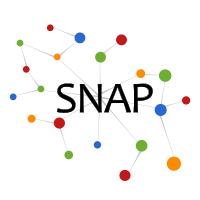Open positions
Open research positions in SNAP group are available here.

# Enhanced Hi-C interaction network

### Dataset information

This is a Hi-C interaction network. Hi-C is a chromosome conformation capture (3C)-based technology, an experimental approach for understanding chromatin interactions within DNA. The Hi-C technology measures pairwise chromatin interaction frequencies within a cell population. The genome is divided into bins of equal size (5K bases long), and the number of contacts observed between each pair of bins is reported. Nodes represent genomic regions and weighted edges represent normalized contacts between two regions in the genome.

Dataset statistics
Nodes (Chromosome 5) 1419
Edges (Chromosome 5) 43763
Nodes in largest SCC (Chromosome 5) 1032
Fraction of nodes in largest SCC (Chromosome 5) 0.727273
Edges in largest SCC (Chromosome 5) 32329
Fraction of edges in largest SCC (Chromosome 5) 0.738729
Average clustering coefficient (Chromosome 5) 0.867585
Number of triangles (Chromosome 5) 1235870
Fraction of closed triangles (Chromosome 5) 0.657262
Diameter (longest shortest path) (Chromosome 5) 10
90-percentile effective diameter (Chromosome 5) 6.561150
Nodes (Chromosome 15) 1164
Edges (Chromosome 15) 35470
Nodes in largest SCC (Chromosome 15) 1054
Fraction of nodes in largest SCC (Chromosome 15) 0.905498
Edges in largest SCC (Chromosome 15) 33018
Fraction of edges in largest SCC (Chromosome 15) 0.930871
Average clustering coefficient (Chromosome 15) 0.874010
Number of triangles (Chromosome 15) 999900
Fraction of closed triangles (Chromosome 15) 0.631299
Diameter (longest shortest path) (Chromosome 15) 9
90-percentile effective diameter (Chromosome 15) 6.088262
Nodes (Chromosome 20) 720
Edges (Chromosome 20) 17972
Nodes in largest SCC (Chromosome 20) 238
Fraction of nodes in largest SCC (Chromosome 20) 0.330556
Edges in largest SCC (Chromosome 20) 6760
Fraction of edges in largest SCC (Chromosome 20) 0.376141
Average clustering coefficient (Chromosome 20) 0.916722
Number of triangles (Chromosome 20) 434618
Fraction of closed triangles (Chromosome 20) 0.649446
Diameter (longest shortest path) (Chromosome 20) 5
90-percentile effective diameter (Chromosome 20) 3.029328

The network is constructed based on the raw contact map, which is normalized and denoised using network enhancement algorithm. Shown are network statistics for three human chromosomes, chromosome 5, 15, and 20.

### Files

File Size Description
GrGr-NE5K.tar.gz 3.5MB Enhanced Hi-C interaction networks and network statistics (one weighted network per human chromosome)